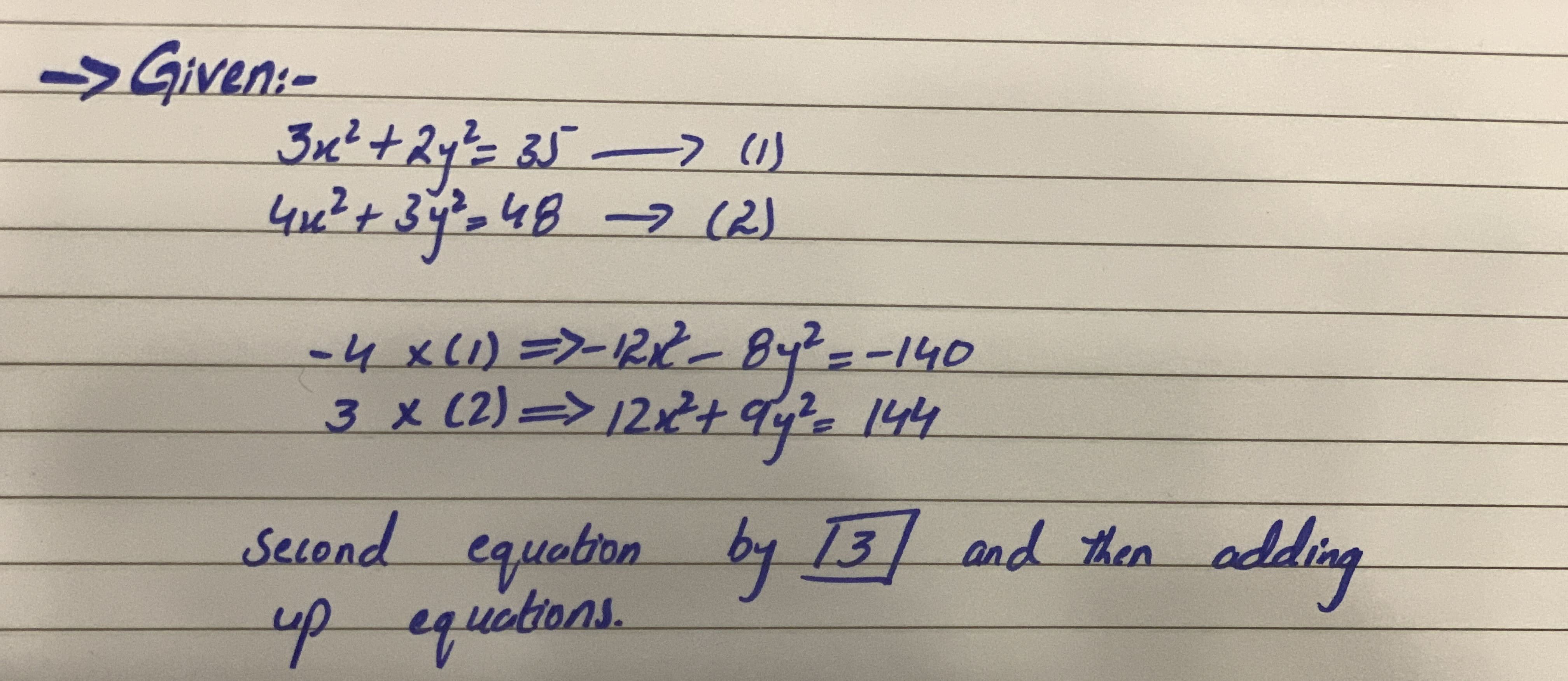# Fill in the bla so the resulting statement is true. when solving 3x^2+2y^2=35 4x^2+3y^2=48 by the addition method, we can eliminate x^2 by the multiplying the first equation by -4 and the second equation by __________ and then adding the equationsamanf 2021-02-14 Answered
Fill in the bla
so the resulting statement is true.
when solving
$3{x}^{2}+2{y}^{2}=35$
$4{x}^{2}+3{y}^{2}=48$
by the addition method, we can eliminate ${x}^{2}$ by the multiplying the first equation by -4 and the second equation by __________ and then adding the equations
You can still ask an expert for help

• Questions are typically answered in as fast as 30 minutes

Solve your problem for the price of one coffee

• Math expert for every subject
• Pay only if we can solve itdessinemoie.............